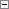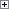Raises a number to a power. For example, you can use this function to square the number 2 to get the result of 4.

NewNumber := POWER(Number, Power)

Parameters

Number

Type: Integer or Decimal

The number you want to raise exponentially. This number is the base in the exponential function.

Power

Type: Integer or Decimal

The exponent in the exponential function.Property Value/Return Value

Type: Decimal

The result of raising Number by Power.Example

This example requires that you create the following variables and text constants in the C/AL Globals window.

Variable name DataType

Number1

Decimal

Power1

Decimal

Result1

Decimal

Text constant name Constant value

Text000

%1 raised to the power of %2 = %3Copy Code
Number1 := 64;
Power1 := 0.5;
Result1 := POWER(Number1, Power1);
MESSAGE(Text000, Number1, Power1, Result1)

On a computer that has the regional format set to English (United States), the first message window displays the following:

64 raised to the power of 0.5 = 8

This example shows that raising a number to the power of 0.5 corresponds to the square root of the number.Example

This example shows a typical use for the POWER function.

If a principal amount P is deposited at interest rate R and compounded annually, then at the end of N years, the accumulated amount (A) is:

A = P(1 + R)N

For example, you put LCY 2800 into a bank account that pays 5 percent, which is compounded quarterly. To determine what the amount will be in eight years, you must consider:

N = 32 payment periods (8 years times 4 quarterly periods)

R = 0.0125 per period (5 percent divided by 4 quarterly periods)

The accumulated amount A is:

A = LCY 2800(1 + 0.0125)32 =LCY 2800(1.4881) = LCY 4166.77

If a principal amount P is deposited at the end of each year at interest rate R (in decimal notation) compounded annually, then at the end of N years, the accumulated amount is:

A = P[((1 + R)N - 1)/R]

This is typically called an annuity.

For example, you have an annuity in which a payment of LCY 500 is made at the end of each year. The interest on this annuity is 4 percent, which is compounded annually. To determine what the annuity will be worth in 20 years, you must consider:

R = 0.04

N = 20

The amount of the annuity A will be:

A = LCY 500[((1 + 0.04)20 - 1)/0.04 = LCY 14,889.04

This example requires that you create the following variables and text constants in the C/AL Globals window.

Variable name DataType

P

Decimal

R

Decimal

N

Decimal

A

Decimal

FormatString

Text

Text constant name Constant value

Text000

Principal \$%1 at a 5 percent interest rate is compounded quarterly.\

Text001

(Rate = %2)\

Text002

The amount after %3 years = \$%4.

Text003

Principal \$%1 is deposited at the end of each year at a 4 percent interest rate, compounded annually.\

Text004

(Rate = %2)\

Text005

The amount after %3 years = \$%4.Copy Code
FormatString := '<Precision,2><Standard Format,1>';
// Example 1
P := 2800;
R := 0.0125;
N := 32;
A = P * (POWER(1 + R, N));
MESSAGE(Text000 + Text001 + Text002, P, R, N, FORMAT(A,0,FormatString);
// Example 2
P = 500;
R = 0.04;
N = 20;
A = P * ((POWER(1 + R, N) - 1)/R);
MESSAGE(Text001, P, R, N, FORMAT(A,0,FormatString));

On a computer that has the regional format set to English (United States), the first message window displays the following:

Principal \$2,800 at a 5 percent interest rate is compounded quarterly.

(Rate = 0.0125)

The amount after 32 years = \$4166.77.

The second message window displays the following:

Principal \$500 is deposited at the end of each year at a 4 percent interest rate, compounded annually.

(Rate = 0.04)

The amount after 20 years = \$14889.04.